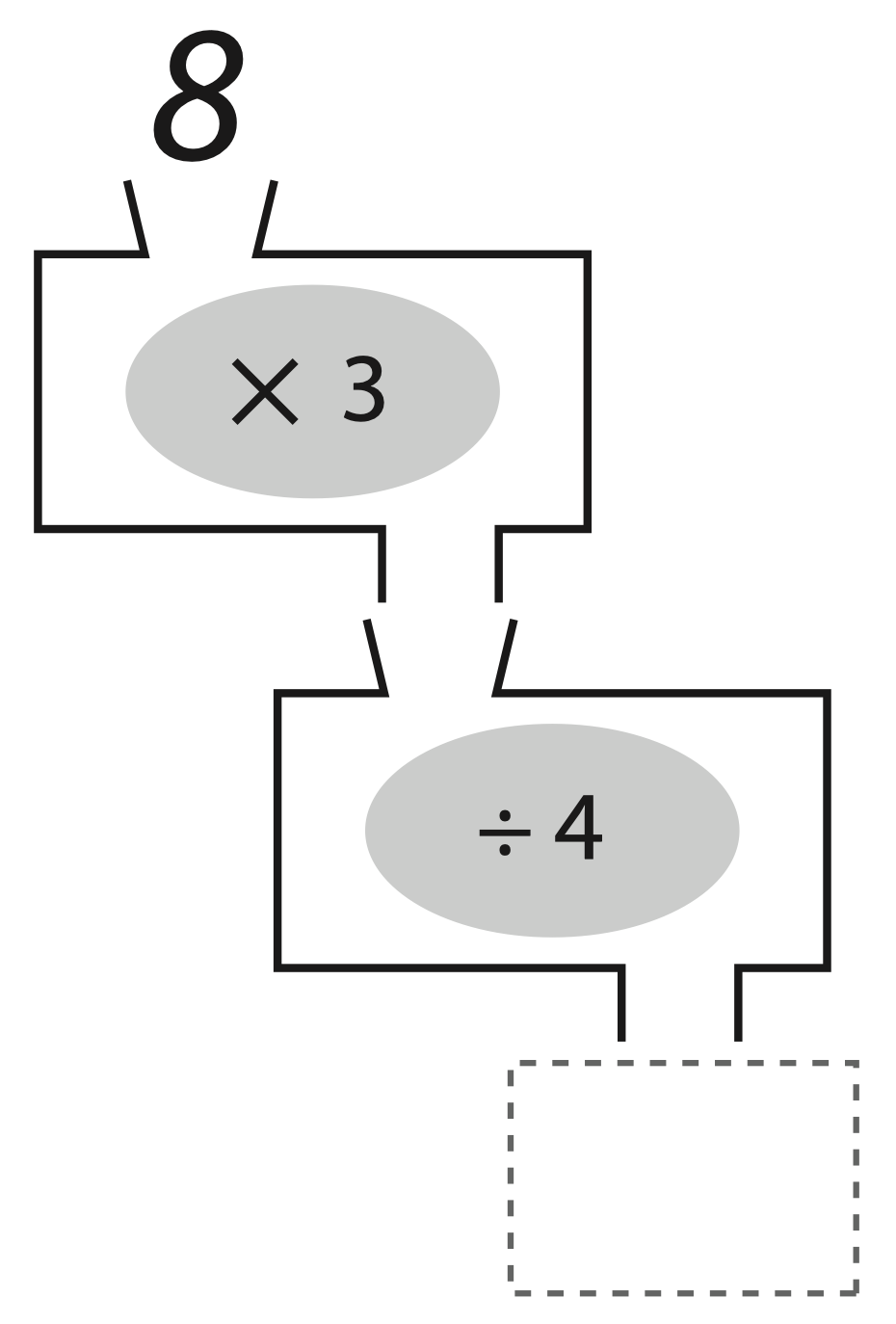I recently helped with a lesson that involved using input/output machines to teach fractions to 5th graders. To help students visualize these machines, I created the animation. It begins with machines that transform shapes into circles to make the idea of the machines more tangible. Then, it switches to math and aligns with some of the first problems in the student work packets for the grade 5 CT lesson. This video can be used in conjunction with the lesson described below or on its own to introduce IO machines and/or functions in programming in a way that is accessible to elementary school students.

## Lesson

I’ve been working with the math coach at my school to incorporate computer science/ computational thinking into elementary school math lessons. One set of lessons we’ve used most recently comes from the Education Development Center. They’ve partnered with the Massachusetts Department of Elementary and Secondary Education to develop a series of lessons, modules, and activities to incorporate computational thinking into existing lessons that align with Massachusetts math and science standards. At the time of this writing, the materials are still under development and may change.

We did a modified version of the Grade 5 Math - Number Fluency and Fractions module. In this module, students use two-step input/output machines as a technique to multiply whole numbers by fractions. For example, $8\times\frac3 4$ can also be expressed as $8\times3\div 4$. We can envision the multiplication and division as two input/output machines. The first machine takes multiplies its input by 3 and the second machine divides its input by 4. The lessons take students through a series of these machines, demonstrating different kinds of algorithms.The lesson plans call for these machines to teach students about algorithms, which help develop computational thinking. They can also set students up to think in terms of functions. In computer science, functions are used to run the same step or steps multiple times without having to define them each time. The function is defined once and called (or run) as many times as necessary. In my experience working with first-time coders in middle and high school, functions can be one of the first major sources of confusion. I’ve been looking for a way to help students visualize functions, and input/output machines are the perfect way to do so.

Tags:

Categories:

Updated:

Get new posts by email: